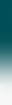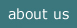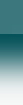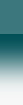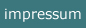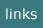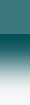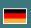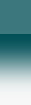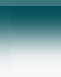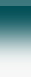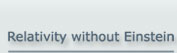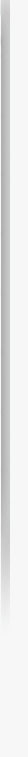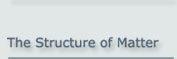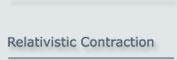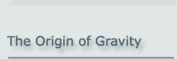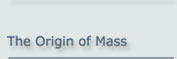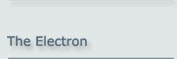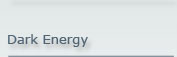The Origin of Relativity

Relativity as founded by Hendrik A. Lorentz follows from the structure of our matterAround the year 1900 several physicists were looking for a physical concept to explain the constancy of the speed of light, which was the result of the famous Michelson-Morley experiment.

Some of them (Lorentz, Heaviside, Lodge, Fitzgerald, Lamor, and Einstein) explained the null effect of the Michelson-Morley experiment by the contraction of matter at movement.

Two of them (Lamor and Einstein) integrated the fact into the theory that there is a different progress of time in different moving frames.

Finally Minkowski replaced the 3+1-dimensional conventional space-and-time system, which uses the Euklidian metrics, by a formal 4-dimensional space-time where time is an imaginary quantity. The combination of Einstein's ideas and the geometry of Minkowksi is now known as the "Einsteinian Interpretation of Relativity".

From today's view the Minkowski formalism is a mathematically elegant way, but it is physically unnecessary and it leads to logical conflicts. The conventional way of the 3+1-dimentional space-and-time using the Euklidian metrics should be kept, but the fact of different clock times in different frames must be integrated. These days this concept is called the "Lorentzian Interpretation of Relativity". It is able to explain all effects of special relativity (SR).

Based on this latter concept, relativity can be understood as the consequence of the internal structure of all elementary particles. The kinematic processes inside an elementary particle are the only reason for the relativistic behavior of matter.

This particle model is presented here. It is compatible with today's experimental state of particle physics.

(Note: This site is also available as a pdf file.)

1. The Concept of the Basic Model of Matter

The concept is:

1. Elementary particles are composed of a pair of "basic particles". The basic particles are bound at a specific distance to each other by a field formation.
2. These basic particles have no mass and orbit each other with the speed of light c.
3. The orbital frequency is the de Broglie frequency.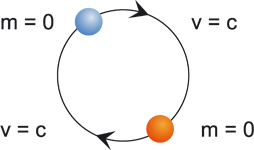Figure 1.1

mass  m = 0

circulation = de Broglie frequency

If it is assumed that every matter is built up in this way, then the well known relativistic effects are a necessary consequence; as well as some observations otherwise explained by quantum mechanics.

That means: Direct consequences of this "basic model" of matter are the principal properties of relativity:

1. If a configuration of basic particles moves, the field binding them contracts by a factor of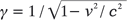As a consequence all objects that are built by basic particles contract
2. The orbital period of the basic particles is extended by the same factor γ. As a consequence the motion of all objects which are built by basic particles is reduced by this factor γ
3. Objects have an inertial mass. This mass increases at movement by the factor γ.

As this model does not need the four-dimensional space-time and uses the conventional Euklidian metrics, it conforms to the Lorentzian interpretation of relativity .

1.1 Reason for Contraction

If two charges are positioned with a displacement r from each other, there is a force effective between both which depends on their displacement. If both charges are moved simultaneously and with a constant displacement this force decreases.

The following figure shows two charges 1 and 2 positioned at distance r by a field formation. This field formation has to be assumed to be caused by a superposition of mono-pole fields, which cause some kind of a multi-pole field. If now the field strength of each single mono-pole field decreases then the resulting field contracts. That means that the potential minimum which defines the position of the charge will move closer to the other charge that causes the field situation, i.e. it will move to a distance of r'.Figure 1.2

Why does the field strength decrease?

By the understanding of today's physics a field is the effect of exchange particles. This view has two consequences:

1. in the case of two moving charges, the path of an exchange particle from one charge to the other one has a different length (i.e. extended or reduced) than if not moving
2. the density of the stream of exchange particles arriving at the other object is changed due to the aberration of the exchange particles.

These effects result in a decrease of the field strength by the Lorentz factor γ

For this result a detailed calculation is available.

Reference if further made here to the work of Lorentz, Heaviside, Lodge, Fitzgerald, as mentioned above, who have worked out at early times that a field of motion contracts.

1.2 Reason for the Dilation of the Rotation Period

The dilation (also called dilatation) can be easily explained by this model as well. If two basic particles are orbiting each other and this circling configuration is as a whole moving along the orbital axis with some speed v, then both basic particles will follow a helical path.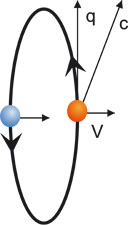Figure 1.3

In the figure above, the orbital speed of the basic particle is now superimposed by the speed v of the whole configuration. The resulting speed of the particle in relation to the reference frame at rest has always to be c . This speed c is now the vector sum of the (reduced) orbital speed q and the speed of the external motion v. So, by the well known relation of Pythagoras, the reduced speed is.

(1.1)

As a consequence, the orbital period T, which is at rest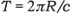.
where R is the radius of the orbit, changes in motion to T':.
Therefore the period is, if compared with the original period, extended by the factor of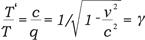(1.2)

If this configuration of two basic particles is moved into a radial rather than an axial direction, there is also an appropriate mathematical deduction of the dilation which is a bit more cumbersome, but the result is similar.

For any arbitrary motion a superposition of an axial and a radial motion can be assumed which leads to the same result. This result is always an extension of the period by the Lorentz factor γ.

1.3 Reason for Mass

The basic particles have no mass. However, there is no doubt that real world objects have mass. So, what is mass? Mass, which here means the inertial mass, is the direct consequence of the finite speed by which any occurrences of a field are propagated.

An elementary particle is built by two basic particles which are bound to each other in a way that the distance r is maintained. If there is a force acting on one of the basic particles, this particle will start to move as a reaction to the force, and the other particle will follow. However, it will not follow instantaneously because it will take the time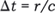(1.3)

until the other particle gets notice of this motion and moves as well. For this time Δt there is still the unchanged binding field of the other particle present with acts against that motion.

To calculate this effect quantitatively it is necessary to know the exact structure of the binding field between both particles.

A field that binds two objects in a way that a distance is maintained will in general have the structure as in the following figure, where the position of object B is defined by the minimum of the field potential caused by object A: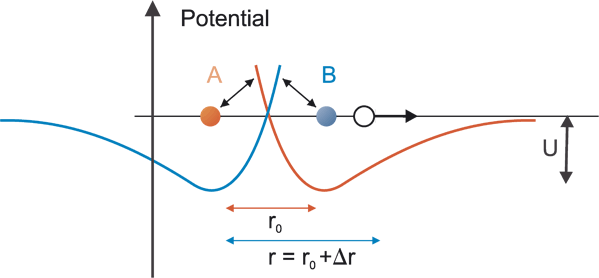Figure 1.4

It will be assumed here that the force is caused by a specific kind of charge which causes an attracting or repelling field. The basic force F between two charged particles with some charge q and a field constant K is assumed to follow the typical dependency of the range: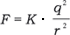(1.4)

The exact curve of this potential field is not known. So we will assume the simplest kind of a multi-pole field for the binding field between both particles: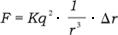(1.5)

where Δr (see fig. 1.4) is the offset to the equilibrium position.

The deduction of this result is presented in detail in the site about the origin of mass.

Now it shall be assumed that one of the particles is accelerated with a constant rate a. The consequence is that, after the initial period Δt, the other particle will also be accelerated by a similar constant rate. However, the acceleration of the other particle will follow the acceleration of the initially accelerated particle with this delay of Δt. So there will be a constant displacement in relation to the equilibrium distance between the particles which results in a constant force between them.

With the acceleration of a, the displacement turns out to be(1.6)

Now, if this is substituted into the formula for F, this yields: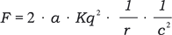(1.7)

The quotient of F and a is, according to the definition of Newton, the inertial mass m:(1.8)

It has, however, to be taken into account that this deduction is only valid for an orientation of the basic particles parallel to the direction of motion which requires the highest amount of force. If the force F is calculated by an integration over all possible directions then the force needed turns out to be reduced to a factor of 4/(i.e. ca. 0.42) of this maximum. This means: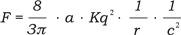and so:(1.9)

This is the inertial mass of an object derived from the delay, by which field forces between charges are propagated.

Several aspects of this result are remarkable. Firstly, it yields the fact that the quotient of force and acceleration is constant at non relativistic velocities. Therefore this is a deduction of Newton’s law of motion. For Newton, this law had the property of an axiom. Secondly, the result shows that the mass is inversely proportional to the size of an elementary particle r.

In the Origin of Mass it is shown that eq. (1.9) is related to the Planck constant in the way:.

(1.10)

If this is substituted in (1.9) and  ħ = h / 2π  is used we get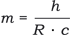(1.11)

or the mass of a particle built by two constituents, where R = r/2 is the radius of the orbit.

The relativistic increase of the mass of a moving body can also be deduced in the way presented here. This is done by taking into account that, in the moving state, the propagation delay of the field and the strength of the field are different and have to be adapted for the motion. If this is done, the derivation as above will end up with a mass which is increased by a factor of γ.

Further we can now use the increase of the mass to derive the famous formula(1.12)

where E is the total energy of the configuration of particles (normally this derivation is done in the opposite direction).

So this famous and important formula is also derived form basic principles, whereas Einstein used the Maxwell theory and a quite demanding gedanken experiment to show that this has to be fulfilled, to avoid contradictions within his physical frame of thought.

1.4 Conclusion

The mathematical deduction shows that the basic model of matter, which is founded on particles with no mass that orbit each other with the speed of light, is able to explain the essential effects of special relativity, namely contraction, dilation of time related processes, and the increase of mass during movement – without any use of the complex four-dimensional space time. Further it explains Newton's law of motion, the conservation of energy and of momentum, and the mass energy equivalence.

Note: The deductions in this chapter are performed in relation to the absolute frame at rest. Measurements made in any different frame of motion in respect to this one will yield identical results. For a proof reference is made here to the literature  regarding the Lorentzian interpretation of special relativity.

In the following, the basic model is used to perform calculations for elementary particles, in order to judge whether the basic model is able to yield quantitatively correct results.

2. Consequences of the Model

2.1 Determination of the Force Binding the Basic Particles

Eq. (1.10) can be written as: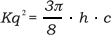(2.1)

Substituting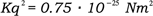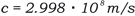results in.
As a comparison, the electrostatic force isSo the value of the force causing the bind of the basic particles is by a factor of 232 greater than the electrostatic force of two elementary charges.

2.2 The Electron as a Test Case for the Model

The electron provides a nice proof for the basic particle model. The basic particle model is able to explain the essential parameters of the electron classically, i.e. without any reference to quantum mechanical calculations which are otherwise understood as unavoidable to understand the electron.

We can easily determine the magnetic momentum of the electron.

The magnetic momentum μ of a loop current is classically: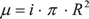(2.2)

The loop current i within the electron is according to the basic particle model: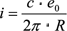(2.3)

so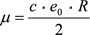(2.4)

If now R is now replaced from eq (1.11) we get purely classically:(2.5)

which is the Bohr magneton.

Note that this is the correct result which does not have the incorrect factor 2 which was the result in early classical calculations when the electron war investigated.

From eq. (1.11) there can be also concluded that the spin of the electron is like for all elementary particles (i.e. leptons and quarks) a constant which is not dependent on the size of the particle.

A detailed deduction of the electron's parameters is available here.

2.3 The Particle Wave Duality (Dualism)

The basic particles are bound to each other by a field. Of course, this field is not only existent in the area between these particles but in every direction around. Since the basic particles are orbiting, this field is an alternating field. It surrounds all elementary particles which are composed by basic particles, and it oscillates according to the assumption of the model with the de Broglie frequency.

If, in a scattering experiment, an elementary particle approaches an obstacle like a double slit, the field surrounding the particle will built an interference structure. This interference structure will guide the elementary particle, because its constituents, the basic particles, are completely destined by this field. So it is clear that, when the elementary particles are registered in some way behind the obstacle, they will not built a shadow-like picture of the obstacle but an interference image. Therefore the experimenting person will have the impression, that not a particle was registered but a wave.

It has, however, to be taken into account that the wavelength effective for the interference result is not directly dependent on the frequency following from this model but on a wavelength which depends on the momentum of the particle, as it was stated by de Broglie in his paper of 1923 . The assumption of de Broglie that this wave has a phase speed greater than c has to be used. Using this assumption the particle scattering can be explained in the scope of the basic particle model.

So this is a way to understand the particle wave duality. (Remark: The picture below is of course not to scale; the diameter of the elementary particle is in practice by several orders of magnitude smaller than the slit.)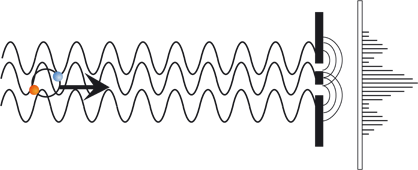Figure 2.3

3. Summary

The "basic model" provides a unification of special relativity and quantum mechanics at least to a certain extent. It is a kind of a generalization of the "Zitterbewegung" of the electron as it was conceived by Erwin Schrödinger.

For relativity it provides an explanation of contraction and dilation in the frame of the Lorentzian interpretation of relativity. For quantum mechanics it provides an explanation of the wave behavior of matter. In this context the basic particles are a representation of the "beables" in the notation of David Bohm.

In present physics special relativity and quantum mechanics are understood to be a quite perfect explanation of physical phenomena for their corresponding field of validity each. The drawback, however, is the fact that it was always found difficult to unify both. The basic model of matter presented here is a fundamental explanation of aspects of both and serves in this way as a unification.

4. Bibliographical References

 Prokhovnik, S. I., The Physical Interpretation of Special Relativity - a Vindication of Hendrik Lorentz. Z. Naturforschung 48a, 925 (1993).

 F. Selleri et al., Die Einsteinsche und lorentzianische Interpretation der speziellen und allgemeinen Relativitätstheorie. Karlsbad:VRI 1998.

 Louis de Broglie, RADIATIONS - Ondes et Quanta, Comptes rendus, Vol. 177, 1923, pp. 507-510.

NOTE:

This concept of the "basic model" of matter was presented initially at the Spring Conference of the German Physical Society (Deutsche Physikalische Gesellschaft) on 24 March 2000 in Dresden

by Dr. Albrecht Giese.

Comments are welcome to note@ag-physics.de.

2009-08-27